# Coordinate Geometry of Straight Lines (E Math)

Author:
Lew W.S.
This book is intended for fostering self directed learning (SDL) of Coordinate Geometry of Straight Lines covered in the Elementary Mathematics syllabus in Singapore for GCE O Level.
•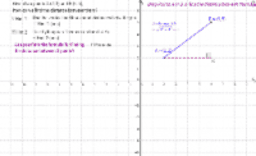### Distance Between Two Points (Length of Line Segment)

•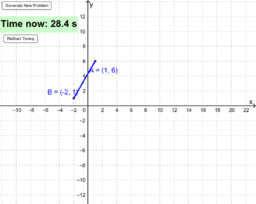### Self Assess Distance Betw Two Points(Length of Line Segment)

•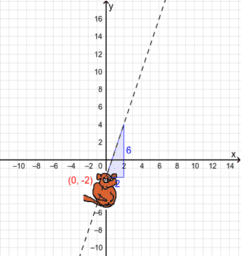### Forming Equation of a Straight Line Given Graph of Line

•### Determining the Equation of Straight Lines Given Two Points

•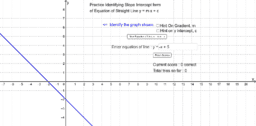### Slope Intercept Form of Equation of Straight Line - Quiz

•### Speed Time Graph for Self Directed Learning (Customizable)

•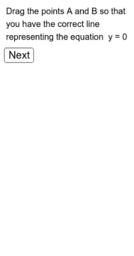### Lines representing equations of the form x = a and y = c

•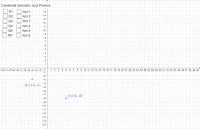### Coordinate Geometry of St Lines Revision Practice

•